# Methods and formulas for the fits and residuals for Stability Study for random batches

Select the method or formula of your choice.

## Fitted value

The predicted y or; the mean response value for the given predictor values using the estimated regression equation.

## Standard error of the marginal fitted value (SE Fit)

The standard error of the marginal fitted values in the mixed model depend on the test method for the fixed effects. For both methods, the standard errors are the square roots of the diagonal elements of the variance matrix of the fits.

where

where

## Residuals

A residual is the difference between an observed value and a fitted value. This part of the observation is not explained by the fitted model. The residual of an observation is:

When batch is a random factor, Minitab calculates 2 types of residuals. Marginal residuals use the fitted value for a random batch, so the coefficient for batch is not in the equation.

Conditional residuals use the fitted value for a batch that is in the data.

### Notation

TermDescription
yiith observed response valueith fitted response value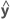the vector of fitted responses
Xthe design matrix for the fixed effects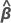the vector of fixed predictors
Zthe design matrix for the random factors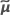the vector of the estimated BLUP values

## Standardized residuals

Standardized residuals are also called "internally Studentized residuals."

where the standard deviation of the residual is the appropriate diagonal square root of the residual variance matrix:

where

### Notation

TermDescription
eithe ith residual
Std(ei)the standard deviation of the ith residual

## Confidence interval

The range in which the estimated mean response for a given set of predictor values is expected to fall.

The standard error of the fitted values in the mixed model are the square roots of the diagonal elements of this matrix:

where

The degrees of freedom use this formula when batch is a random factor:

where

### Notation

TermDescription
t1-α/2, df1–α/2 quantile from the t distribution with the given degrees of freedom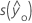standard error of the fitted value
Xdesign matrix, including the constant
X' transpose of X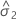variance component for error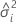variance component of the ith random factor
Zin x mi matrix of known codings for the ith random effect in the model
Zi' transpose of Zi
Inidentity matrix with n rows and columns
xi predictor values for the fit or prediction
W asymptotic variance-covariance matrix of the variance component for error
cnumber of random effects in the model

## Prediction interval

The range in which the predicted response for a new observation is expected to fall. The calculation of the prediction interval depends on whether you compute the interval for the marginal fit or for the conditional fit.

### Marginal fit

where

The degrees of freedom for the t-statistic are given by this formula:

where

### Conditional fit

where

The degrees of freedom for the t-statistic are:

where

### Notation

TermDescription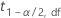1–α/2 quantile from the t distribution with the given degrees of freedom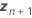vector of the new values of the random predictorsvariance component for error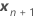vector of new values of the fixed predictorsvariance component of the ith random factor
Im identity matrix with m rows and columns
mnumber of columns in the design matrix to represent the ith random term in the model
c number of random effects in the model
Zin x mi design matrix for the ith random effect in the model
Z'itranspose of Zi
By using this site you agree to the use of cookies for analytics and personalized content.  Read our policy